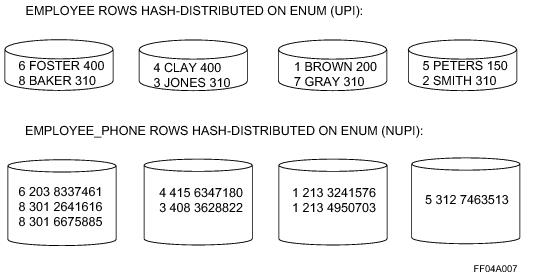# 15.10 - Merge Join - Teradata Database

## Teradata Database SQL Request and Transaction Processing

prodname
vrm_release
15.10
category
Programming Reference
User Guide
featnum
B035-1142-151K

A merge join retrieves rows from two tables by processing equality joins that probe one of the tables using either row key or row hash values from the qualified rows in the other table being joined. A merge join assumes that the probed table is sorted either by row key or row hash. The operation then puts the joined rows onto a common AMP based on the row key or row hash of the join columns. Teradata Database sorts the rows into join column row key or row hash sequence, then joins those rows that have matching join column row key or row hash values.

In a merge join, the columns on which tables are matched are also the columns on which both tables, or redistributed spools of tables, are ordered. Merge join is generally more efficient than a product join (see “Product Join” on page 398) because it requires fewer comparisons and because blocks from both tables are read only once.

Two different general merge join algorithms are available.

• Slow path merge join (see “Slow Path Inner Merge Join” on page 409)
• A merge join algorithm in which one of the tables is accessed using an index. All of the rows returned by means of the access path are joined without using a rowhash match scan.

The slow path is used when the left table is accessed using a read mode other than an all-rows scan. The determination is made in the AMP, not by the Optimizer.

• Fast path merge join (see “Fast Path Inner Merge Join” on page 409)
• A merge join algorithm in which an index access path is not used on either table. Matching rows are joined using a rowhash match scan.

The fast path is used when the left table is accessed using the all-row scan reading mode.

Each of these general merge join algorithms can also be applied to the various merge join methods. Geospatial column terms are not permitted for outer join conditions.

• Fast path inner merge join (see “Fast Path Inner Merge Join” on page 409)
• Fast path inner inclusion merge join (see “Inclusion Merge Join Process” on page 467)

• Slow path inner merge join (see “Slow Path Inner Merge Join” on page 409)
• Slow path inner inclusion merge join (see “Inclusion Merge Join Process” on page 467)

• Exclusion merge join (see “Exclusion Merge Join” on page 463)
• Fast path left outer join
• Fast path left outer inclusion merge join (see “Inclusion Merge Join Process” on page 467).

• Slow path left outer join
• Slow path left outer inclusion merge join (see “Inclusion Merge Join Process” on page 467).

• Fast path right outer merge join
• Slow path right outer merge join
• Full outer merge join
• Another class of merge join methods is used only with row-partitioned tables.

• Direct merge join (see “Direct Row-partitioned PI Merge Join” on page 415)
• Rowkey‑based merge join (see “Rowkey-Based Merge Join” on page 417)
• Single window merge join (see “Single‑Window Merge Join” on page 425)
• Sliding window merge join (see “Sliding‑Window Merge Join” on page 428)
• Dynamic row partition elimination (see “Product Joins With Dynamic Row Partition Elimination” on page 400 and Database Design) is not supported for merge joins of MLPPI tables.

The Optimizer has three general approaches when joining a row-partitioned PI table to a nonpartitioned table or when joining two row-partitioned PI tables with different partitioning expressions.

• Spool the row-partitioned PI table (or both row-partitioned PI tables) into a nonpartitioned spool in preparation for a traditional merge join.
• Spool the nonpartitioned table (or one of the two row-partitioned PI tables) into a row-partitioned PI spool, with identical partitioning to the remaining table, in preparation for a rowkey-based merge join (see “Rowkey-Based Merge Join” on page 417).
• This option is not always available.

• Use the sliding window merge join of the tables without spooling either one (see “Sliding‑Window Merge Join” on page 428).
• In this case, both tables must have the same primary index and the join must be an equality join between the primary index columns and neither can be column-partitioned.

In all cases, the Optimizer considers all reasonable join strategies and selects the one with the least estimated cost.

Note: The number of partition contexts per row-partitioned PI table when joining the table either to another row-partitioned PI table or to a non‑PPI table ranges between 2 and 8, depending on the table block size and the size of the FSG cache.

Depending on the relative costs, the Optimizer might substitute a form of hash join (see “Hash Join” on page 434 and “Dynamic Hash Joins” on page 443) in place of a merge join when there is no skew in the data, depending on the relative costs of the two methods.

The high level process applied by the merge join algorithm is the following:

1 Identify the smaller relation of the pair to be joined.

2 The Optimizer pursues the following steps only if it is necessary to place qualified rows into a spool.

a Place the qualifying rows from one or both relations into separate spools.

As the qualified rows are placed into spool, relocate them to their target AMPs based on the hash of the join column set.

b Sort the qualified spool rows on their join column row hash values.

3 Compare the relocated row set with matching join column row hash values in the other relation.

To cost the possible binary combinations of merge join strategies, the following combinations of relations R1 and R2 are analyzed:

• R1 as the left relation, R2 as the right relation using the fast path method.
• R1 as the left relation, R2 as the right relation using the slow path method.
• This is only costed if there is an access path for R1.

• R2 as the left relation, R1 as the right relation using the fast path method.
• R2 as the left relation, R1 as the right relation using the slow path method.
• This is only costed if there is an access path for R2.

The Optimizer selects the least costly combination to be used for further comparisons.

When there are equality join terms on all primary index columns of a single‑level row-partitioned PI table plus certain forms of range join terms on the partitioning column, then the Optimizer might use a merge join with dynamic row partition elimination to make the join.

You can think of this as a form of sliding window merge join (see “Sliding‑Window Merge Join” on page 428) with the row-partitioned PI table in which the other table being joined is partitioned the same way as the row-partitioned PI table, and a subset of the partitions in one table is joined to a subset of the row partitions in the other table as determined by the specified range terms.

Dynamic row partition elimination-applicable join terms must be specified in one the following forms.

• ppi_t1.partitioning_column_1 + C1 <operator> ppi_t2. partitioning_column_2
• ppi_t2. partitioning_column_2 <operator> ppi_t1.partitioning_column_1 + C2
• ppi_t1.partitioning_column_1 + C1 <operator> ppi_t2. partitioning_column_2
• ppi_t2.non_partitioning_column_2 <operator> ppi_t1.ppi_column_1 + C2
• where:

 Syntax element … Specifies … one of the following join operators. < <= >= > ppi_t1.partitioning_column_1 a partitioning column for table ppi_t1. C1 a constant. ppi_t2. partitioning_column_2 a partitioning column for table ppi_t2. C2 a constant. ppi_t1.ppi_column a partitioning column for table ppi_t1. ppi_t2.non_partitioning_column a partitioning column for table ppi_t2.

Because you cannot arithmetically add strings together, and because string intervals are not defined, merge join dynamic row partition elimination is not supported for character-partitioned tables. As a result, the Optimizer never selects this join method as a direct join to a character-partitioned table.

For most cases, the rows of a table that does not have a primary [AMP] index on which the equality join is specified require a change in their geography before they can be merge joined with a primary‑[AMP-]indexed table.

The only time it is possible to avoid relocating these rows across the AMPs is the case where the other table is duplicated. In all cases documented in the following table, the assumption is made that the hashes needed for making the join are preserved when the demographics for the rows change.

 Merge Join Demographics Merge Join Demographics Table Direct Table Local Table Duplicated Table Redistributed PI Table Direct Not valid Not valid Valid Valid PI Table Local Not valid Not valid Valid Valid PI Table Duplicated Not valid Valid Not valid Not valid PI Table Redistributed Not valid Not valid Not valid Valid

The process applied by the slow path merge join algorithm is as follows.

1 Read each row from the left table.

2 Join each left table row with the right table rows having the same hash value.

The following graphic illustrates the generic slow path merge join process.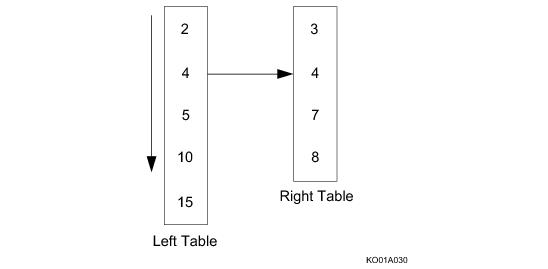The process applied by the fast path merge join algorithm is as follows.

1 Read a row from the left table and record its hash value.

2 Read the next row from the right table that has a row hash >= to that of the left table row.

 IF the row hash values are … THEN … equal join the two rows. not equal use the larger row hash value to read the row from the other table.

The following graphic illustrates the generic fast path merge join process: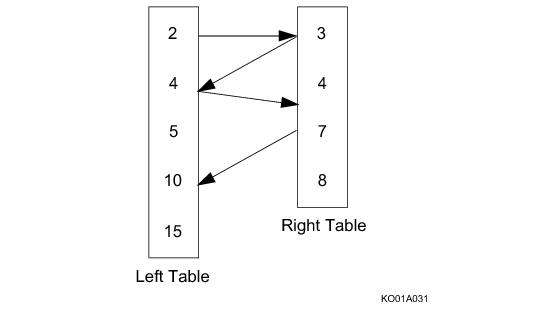Merge joins use one of the following distribution strategies:

 Strategy Number Strategy Name Stage Process 1 Hash Redistribution 1 Hash redistribute one or both sides to few or all AMPs (depending on the primary indexes used in the join). If a table has a Primary AMP on the join columns, Teradata Database can spool locally instead of using a hash redistribution, but stage 2 for the table is still needed. 2 Sort the rows into join column row hash sequence. 2 Duplication 1 Duplicate the smaller side on all AMPs. 2 Sort the rows into the row hash sequence of the join column. 3 Locally copy the other side and sort the rows into row hash sequence of the join column. 3 Index Matching 1 No redistribution is required if the primary indexes are the join columns and if they match.

 FOR a representation of this strategy … See this illustration … Hash redistribution Duplication Index matching

The following SELECT request determines who works in what location by merge joining the employee and department tables on the condition that the dept_no values in both tables are equal.

`     SELECT name, dept_name, loc `
`     FROM employee INNER JOIN department`
`     WHERE employee.dept_no = department.dept_no;`

The following list presents the stages of processing this merge join.

1 Because department rows are distributed according to the hash code for dept_no (the unique primary index of the department table), employee rows are themselves redistributed by the hash code of their own dept_no values.

2 The redistributed employee rows are stored in a spool on each AMP and sorted by the hash value for dept_no.

This puts them in the same order as the department rows on the AMP.

3 The hash value of the first row from the department table will be used to read the first row with the same or bigger row hash from the employee spool.

That is, rows from either table of a merge join are skipped where possible.

4 If there is a hash codes match, an additional test is performed to verify that the matched rows are equivalent in dept_no value as well as hash value.

5 If there is no hash code match, then the larger of the two hash codes is used to position to the other table.

The hash code and value comparisons continue until the end of one of the tables is reached.

6 On each AMP the name, dept_no, and loc values from each of the qualifying rows are placed in a result spool.

7 When the last AMP has completed its merge join, the contents of all result spools are merged and returned to the user.

When many rows fail to meet a constraint, the hash‑match‑reposition process might skip several rows. Skipping disqualified rows can speed up the merge join execution, especially if the tables are very large.

The following graphic illustrates this merge join process: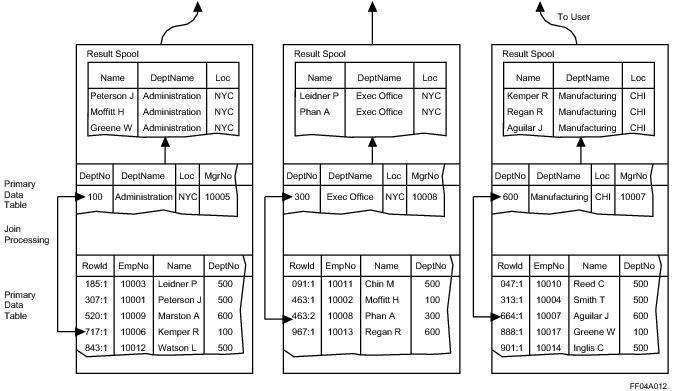The next merge join example uses the following table definitions.

One of the merge join row redistribution methods (see “Merge Join Row Distribution (Hash Redistribution)” on page 413 or “Merge Join Row Distribution (Duplicate Table)” on page 413) is used if you perform the following SELECT request against these tables.

`   SELECT *`
`   FROM employee INNER JOIN department`
`   WHERE employee.dept = department.dept;`

The following graphic shows a merge join row distribution using hash redistribution: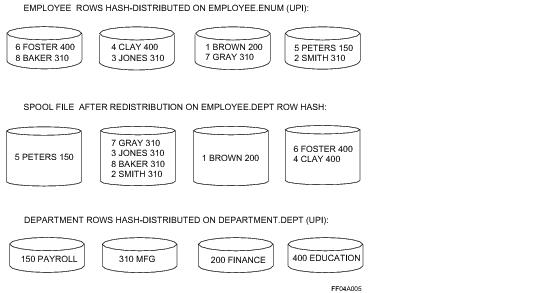The following graphic shows a merge join row distribution by duplicating a table.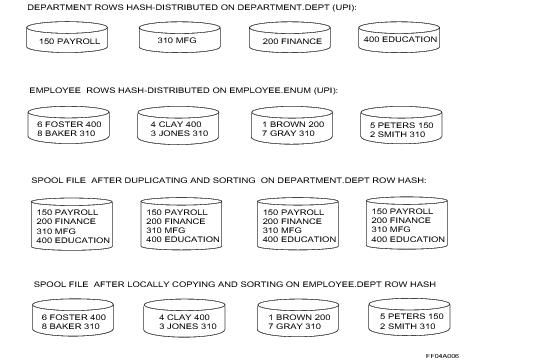This example uses the following employee and employee_phone table definitions.

The matching indexes row distribution strategy shown in “Merge Join Row Distribution Using Index Matching” on page 414 is used if you perform the following SELECT request against these tables.

`   SELECT *`
`   FROM employee INNER JOIN employee_phone`
`   WHERE employee.e_num = employee_phone.e_num;`

The following graphic shows a merge join row distribution by matching indexes: